Zeros of Polynomial Functions

LEARNING OBJECTIVES

By the end of this lesson, you will be able to:

• Evaluate a polynomial using the Remainder Theorem.
• Use the Factor Theorem to solve a polynomial equation.
• Use the Rational Zero Theorem to find rational zeros.
• Find zeros of a polynomial function.
• Use the Linear Factorization Theorem to find polynomials with given zeros.
• Use Descartes’ Rule of Signs.
• Solve real-world applications of polynomial equations

A new bakery offers decorated sheet cakes for children’s birthday parties and other special occasions. The bakery wants the volume of a small cake to be 351 cubic inches. The cake is in the shape of a rectangular solid. They want the length of the cake to be four inches longer than the width of the cake and the height of the cake to be one-third of the width. What should the dimensions of the cake pan be?

This problem can be solved by writing a cubic function and solving a cubic equation for the volume of the cake. In this section, we will discuss a variety of tools for writing polynomial functions and solving polynomial equations.

Evaluate a polynomial using the Remainder Theorem

In the last section, we learned how to divide polynomials. We can now use polynomial division to evaluate polynomials using the Remainder Theorem. If the polynomial is divided by x – k, the remainder may be found quickly by evaluating the polynomial function at k, that is, f(k) Let’s walk through the proof of the theorem.

Recall that the Division Algorithm states that, given a polynomial dividend f(x) and a non-zero polynomial divisor d(x) where the degree of d(x) is less than or equal to the degree of f(x), there exist unique polynomials q(x) and r(x) such that

$f\left(x\right)=d\left(x\right)q\left(x\right)+r\left(x\right)$

If the divisor, d(x), is x – k, this takes the form

$f\left(x\right)=\left(x-k\right)q\left(x\right)+r$

Since the divisor x – k is linear, the remainder will be a constant, r. And, if we evaluate this for xk, we have

$\begin{cases}f\left(k\right)=\left(k-k\right)q\left(k\right)+r\hfill \\ \text{ }=0\cdot q\left(k\right)+r\hfill \\ \text{ }=r\hfill \end{cases}$

In other words, f(k) is the remainder obtained by dividing f(x) by x – k.

A General Note: The Remainder Theorem

If a polynomial $f\left(x\right)$ is divided by x – k, then the remainder is the value $f\left(k\right)$.

How To: Given a polynomial function $f$, evaluate $f\left(x\right)$ at $x=k$ using the Remainder Theorem.

1. Use synthetic division to divide the polynomial by $x-k$.
2. The remainder is the value $f\left(k\right)$.

Example 1: Using the Remainder Theorem to Evaluate a Polynomial

Use the Remainder Theorem to evaluate $f\left(x\right)=6{x}^{4}-{x}^{3}-15{x}^{2}+2x - 7$ at $x=2$.

Solution

To find the remainder using the Remainder Theorem, use synthetic division to divide the polynomial by $x - 2$.

$\begin{cases}\\ 2\overline{)\begin{cases}6\hfill & -1\hfill & -15\hfill & 2\hfill & -7\hfill \\ \hfill & 12\hfill & \text{ }22\hfill & 14\hfill & 32\hfill \end{cases}}\\ \begin{cases}\text{ }6\hfill & 11\hfill & \text{ }7\hfill & \text{16}\hfill & 25\hfill \end{cases}\end{cases}$

The remainder is 25. Therefore, $f\left(2\right)=25$.

Analysis of the Solution

We can check our answer by evaluating $f\left(2\right)$.

$\begin{cases}f\left(x\right) & =6{x}^{4}-{x}^{3}-15{x}^{2}+2x - 7 \\ f\left(2\right) & =6{\left(2\right)}^{4}-{\left(2\right)}^{3}-15{\left(2\right)}^{2}+2\left(2\right)-7 \\ \hfill & =25\hfill \end{cases}$

Try It 1

Use the Remainder Theorem to evaluate $f\left(x\right)=2{x}^{5}-3{x}^{4}-9{x}^{3}+8{x}^{2}+2$
at $x=-3$.

Solution

Use the Factor Theorem to solve a polynomial equation

The Factor Theorem is another theorem that helps us analyze polynomial equations. It tells us how the zeros of a polynomial are related to the factors. Recall that the Division Algorithm tells us

$f\left(x\right)=\left(x-k\right)q\left(x\right)+r$.

If k is a zero, then the remainder r is $f\left(k\right)=0$ and $f\left(x\right)=\left(x-k\right)q\left(x\right)+0$ or $f\left(x\right)=\left(x-k\right)q\left(x\right)$.

Notice, written in this form, x – k is a factor of $f\left(x\right)$. We can conclude if is a zero of $f\left(x\right)$, then $x-k$ is a factor of $f\left(x\right)$.

Similarly, if $x-k$ is a factor of $f\left(x\right)$, then the remainder of the Division Algorithm $f\left(x\right)=\left(x-k\right)q\left(x\right)+r$ is 0. This tells us that k is a zero.

This pair of implications is the Factor Theorem. As we will soon see, a polynomial of degree n in the complex number system will have n zeros. We can use the Factor Theorem to completely factor a polynomial into the product of n factors. Once the polynomial has been completely factored, we can easily determine the zeros of the polynomial.

A General Note: The Factor Theorem

According to the Factor Theorem, k is a zero of $f\left(x\right)$ if and only if $\left(x-k\right)$ is a factor of $f\left(x\right)$.

How To: Given a factor and a third-degree polynomial, use the Factor Theorem to factor the polynomial.

1. Use synthetic division to divide the polynomial by $\left(x-k\right)$.
2. Confirm that the remainder is 0.
3. Write the polynomial as the product of $\left(x-k\right)$ and the quadratic quotient.
4. If possible, factor the quadratic.
5. Write the polynomial as the product of factors.

Example 2: Using the Factor Theorem to Solve a Polynomial Equation

Show that $\left(x+2\right)$ is a factor of ${x}^{3}-6{x}^{2}-x+30$. Find the remaining factors. Use the factors to determine the zeros of the polynomial.

Solutions

We can use synthetic division to show that $\left(x+2\right)$ is a factor of the polynomial.

The remainder is zero, so $\left(x+2\right)$ is a factor of the polynomial. We can use the Division Algorithm to write the polynomial as the product of the divisor and the quotient:

$\left(x+2\right)\left({x}^{2}-8x+15\right)$

We can factor the quadratic factor to write the polynomial as

$\left(x+2\right)\left(x - 3\right)\left(x - 5\right)$

By the Factor Theorem, the zeros of ${x}^{3}-6{x}^{2}-x+30$ are –2, 3, and 5.

Try It 2

Use the Factor Theorem to find the zeros of $f\left(x\right)={x}^{3}+4{x}^{2}-4x - 16$ given that $\left(x - 2\right)$ is a factor of the polynomial.

Solution

Use the Rational Zero Theorem to find rational zeros

Another use for the Remainder Theorem is to test whether a rational number is a zero for a given polynomial. But first we need a pool of rational numbers to test. The Rational Zero Theorem helps us to narrow down the number of possible rational zeros using the ratio of the factors of the constant term and factors of the leading coefficient of the polynomial

Consider a quadratic function with two zeros, $x=\frac{2}{5}$ and $x=\frac{3}{4}$.

By the Factor Theorem, these zeros have factors associated with them. Let us set each factor equal to 0, and then construct the original quadratic function absent its stretching factor.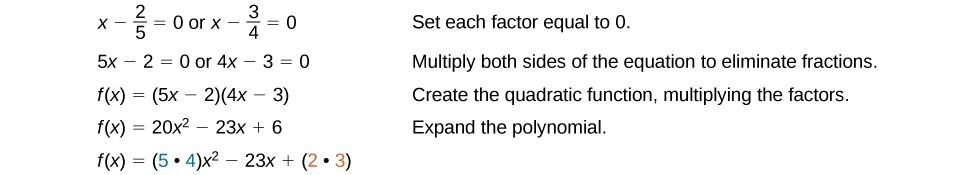Notice that two of the factors of the constant term, 6, are the two numerators from the original rational roots: 2 and 3. Similarly, two of the factors from the leading coefficient, 20, are the two denominators from the original rational roots: 5 and 4.

We can infer that the numerators of the rational roots will always be factors of the constant term and the denominators will be factors of the leading coefficient. This is the essence of the Rational Zero Theorem; it is a means to give us a pool of possible rational zeros.

A General Note: The Rational Zero Theorem

The Rational Zero Theorem states that, if the polynomial $f\left(x\right)={a}_{n}{x}^{n}+{a}_{n - 1}{x}^{n - 1}+…+{a}_{1}x+{a}_{0}$ has integer coefficients, then every rational zero of $f\left(x\right)$ has the form $\frac{p}{q}$ where p is a factor of the constant term ${a}_{0}$ and q is a factor of the leading coefficient ${a}_{n}$.

When the leading coefficient is 1, the possible rational zeros are the factors of the constant term.

How To: Given a polynomial function $f\left(x\right)$, use the Rational Zero Theorem to find rational zeros.

1. Determine all factors of the constant term and all factors of the leading coefficient.
2. Determine all possible values of $\frac{p}{q}$, where p is a factor of the constant term and q is a factor of the leading coefficient. Be sure to include both positive and negative candidates.
3. Determine which possible zeros are actual zeros by evaluating each case of $f\left(\frac{p}{q}\right)$.

Example 3: Listing All Possible Rational Zeros

List all possible rational zeros of $f\left(x\right)=2{x}^{4}-5{x}^{3}+{x}^{2}-4$.

Solution

The only possible rational zeros of $f\left(x\right)$ are the quotients of the factors of the last term, –4, and the factors of the leading coefficient, 2.

The constant term is –4; the factors of –4 are $p=\pm 1,\pm 2,\pm 4$.

The leading coefficient is 2; the factors of 2 are $q=\pm 1,\pm 2$.

If any of the four real zeros are rational zeros, then they will be of one of the following factors of –4 divided by one of the factors of 2.

$\begin{cases}\frac{p}{q}=\pm \frac{1}{1},\pm \frac{1}{2}\text{ }& \frac{p}{q}=\pm \frac{2}{1},\pm \frac{2}{2}\text{ }& \frac{p}{q}=\pm \frac{4}{1},\pm \frac{4}{2}\end{cases}$

Note that $\frac{2}{2}=1$ and $\frac{4}{2}=2$, which have already been listed. So we can shorten our list.

$\frac{p}{q}=\frac{\text{Factors of the last}}{\text{Factors of the first}}=\pm 1,\pm 2,\pm 4,\pm \frac{1}{2}$

Example 4: Using the Rational Zero Theorem to Find Rational Zeros

Use the Rational Zero Theorem to find the rational zeros of $f\left(x\right)=2{x}^{3}+{x}^{2}-4x+1$.

Solution

The Rational Zero Theorem tells us that if $\frac{p}{q}$ is a zero of $f\left(x\right)$, then p is a factor of 1 and q is a factor of 2.

$\begin{cases}\frac{p}{q}=\frac{\text{factor of constant term}}{\text{factor of leading coefficient}}\hfill \\ \text{ }=\frac{\text{factor of 1}}{\text{factor of 2}}\hfill \end{cases}$

The factors of 1 are $\pm 1$ and the factors of 2 are $\pm 1$ and $\pm 2$. The possible values for $\frac{p}{q}$ are $\pm 1$ and $\pm \frac{1}{2}$. These are the possible rational zeros for the function. We can determine which of the possible zeros are actual zeros by substituting these values for x in $f\left(x\right)$.

$\begin{cases}\text{ }f\left(-1\right)=2{\left(-1\right)}^{3}+{\left(-1\right)}^{2}-4\left(-1\right)+1=4\hfill \\ \text{ }f\left(1\right)=2{\left(1\right)}^{3}+{\left(1\right)}^{2}-4\left(1\right)+1=0\hfill \\ \text{ }f\left(-\frac{1}{2}\right)=2{\left(-\frac{1}{2}\right)}^{3}+{\left(-\frac{1}{2}\right)}^{2}-4\left(-\frac{1}{2}\right)+1=3\hfill \\ \text{ }f\left(\frac{1}{2}\right)=2{\left(\frac{1}{2}\right)}^{3}+{\left(\frac{1}{2}\right)}^{2}-4\left(\frac{1}{2}\right)+1=-\frac{1}{2}\hfill \end{cases}$

Of those, $-1,-\frac{1}{2},\text{ and }\frac{1}{2}$ are not zeros of $f\left(x\right)$. 1 is the only rational zero of $f\left(x\right)$.

Try It 3

Use the Rational Zero Theorem to find the rational zeros of $f\left(x\right)={x}^{3}-5{x}^{2}+2x+1$.

Solution

Find zeros of a polynomial function

The Rational Zero Theorem helps us to narrow down the list of possible rational zeros for a polynomial function. Once we have done this, we can use synthetic division repeatedly to determine all of the zeros of a polynomial function.

How To: Given a polynomial function $f$, use synthetic division to find its zeros.

1. Use the Rational Zero Theorem to list all possible rational zeros of the function.
2. Use synthetic division to evaluate a given possible zero by synthetically dividing the candidate into the polynomial. If the remainder is 0, the candidate is a zero. If the remainder is not zero, discard the candidate.
3. Repeat step two using the quotient found with synthetic division. If possible, continue until the quotient is a quadratic.
4. Find the zeros of the quadratic function. Two possible methods for solving quadratics are factoring and using the quadratic formula.

Example 5: Finding the Zeros of a Polynomial Function with Repeated Real Zeros

Find the zeros of $f\left(x\right)=4{x}^{3}-3x - 1$.

Solution

The Rational Zero Theorem tells us that if $\frac{p}{q}$ is a zero of $f\left(x\right)$, then is a factor of –1 and q is a factor of 4.

$\begin{cases}\frac{p}{q}=\frac{\text{factor of constant term}}{\text{factor of leading coefficient}}\hfill \\ \text{ }=\frac{\text{factor of -1}}{\text{factor of 4}}\hfill \end{cases}$

The factors of –1 are $\pm 1$ and the factors of 4 are $\pm 1,\pm 2$, and $\pm 4$. The possible values for $\frac{p}{q}$ are $\pm 1,\pm \frac{1}{2}$, and $\pm \frac{1}{4}$.
These are the possible rational zeros for the function. We will use synthetic division to evaluate each possible zero until we find one that gives a remainder of 0. Let’s begin with 1.

Dividing by $\left(x - 1\right)$ gives a remainder of 0, so 1 is a zero of the function. The polynomial can be written as

$\left(x - 1\right)\left(4{x}^{2}+4x+1\right)$.

The quadratic is a perfect square. $f\left(x\right)$ can be written as

$\left(x - 1\right){\left(2x+1\right)}^{2}$.

We already know that 1 is a zero. The other zero will have a multiplicity of 2 because the factor is squared. To find the other zero, we can set the factor equal to 0.

$\begin{cases}2x+1=0\hfill \\ \text{ }x=-\frac{1}{2}\hfill \end{cases}$

The zeros of the function are 1 and $-\frac{1}{2}$ with multiplicity 2.

Analysis of the Solution

Look at the graph of the function f in Figure 1. Notice, at $x=-0.5$, the graph bounces off the x-axis, indicating the even multiplicity (2,4,6…) for the zero –0.5. At $x=1$, the graph crosses the x-axis, indicating the odd multiplicity (1,3,5…) for the zero $x=1$.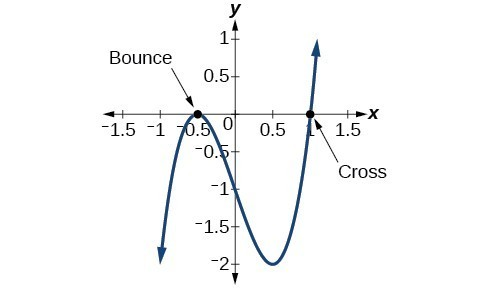Figure 1

Use the Fundamental Theorem of Algebra

Now that we can find rational zeros for a polynomial function, we will look at a theorem that discusses the number of complex zeros of a polynomial function. The Fundamental Theorem of Algebra tells us that every polynomial function has at least one complex zero. This theorem forms the foundation for solving polynomial equations.

Suppose f is a polynomial function of degree four, and $f\left(x\right)=0$. The Fundamental Theorem of Algebra states that there is at least one complex solution, call it ${c}_{1}$. By the Factor Theorem, we can write $f\left(x\right)$ as a product of $x-{c}_{\text{1}}$ and a polynomial quotient. Since $x-{c}_{\text{1}}$ is linear, the polynomial quotient will be of degree three. Now we apply the Fundamental Theorem of Algebra to the third-degree polynomial quotient. It will have at least one complex zero, call it ${c}_{\text{2}}$. So we can write the polynomial quotient as a product of $x-{c}_{\text{2}}$ and a new polynomial quotient of degree two. Continue to apply the Fundamental Theorem of Algebra until all of the zeros are found. There will be four of them and each one will yield a factor of $f\left(x\right)$.

A General Note: The Fundamental Theorem of Algebra states that, if f(x) is a polynomial of degree n > 0, then f(x) has at least one complex zero.

We can use this theorem to argue that, if $f\left(x\right)$ is a polynomial of degree $n>0$, and a is a non-zero real number, then $f\left(x\right)$ has exactly n linear factors

$f\left(x\right)=a\left(x-{c}_{1}\right)\left(x-{c}_{2}\right)…\left(x-{c}_{n}\right)$

where ${c}_{1},{c}_{2},…,{c}_{n}$ are complex numbers. Therefore, $f\left(x\right)$ has n roots if we allow for multiplicities.

Q & A

Does every polynomial have at least one imaginary zero?

No. A complex number is not necessarily imaginary. Real numbers are also complex numbers.

Example 6: Finding the Zeros of a Polynomial Function with Complex Zeros

Find the zeros of $f\left(x\right)=3{x}^{3}+9{x}^{2}+x+3$.

Solution

The Rational Zero Theorem tells us that if $\frac{p}{q}$ is a zero of $f\left(x\right)$, then p is a factor of 3 and q is a factor of 3.

$\begin{cases}\frac{p}{q}=\frac{\text{factor of constant term}}{\text{factor of leading coefficient}}\hfill \\ \text{ }=\frac{\text{factor of 3}}{\text{factor of 3}}\hfill \end{cases}$

The factors of 3 are $\pm 1$ and $\pm 3$. The possible values for $\frac{p}{q}$, and therefore the possible rational zeros for the function, are $\pm 3,\text{\pm 1, and }\pm \frac{1}{3}$. We will use synthetic division to evaluate each possible zero until we find one that gives a remainder of 0. Let’s begin with –3.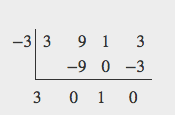Dividing by $\left(x+3\right)$ gives a remainder of 0, so –3 is a zero of the function. The polynomial can be written as

$\left(x+3\right)\left(3{x}^{2}+1\right)$

We can then set the quadratic equal to 0 and solve to find the other zeros of the function.

$\begin{cases}3{x}^{2}+1=0\hfill \\ \text{ }{x}^{2}=-\frac{1}{3}\hfill \\ \text{ }x=\pm \sqrt{-\frac{1}{3}}=\pm \frac{i\sqrt{3}}{3}\hfill \end{cases}$

The zeros of $f\left(x\right)$ are –3 and $\pm \frac{i\sqrt{3}}{3}$.

Analysis of the Solution

Look at the graph of the function f. Notice that, at $x=-3$, the graph crosses the x-axis, indicating an odd multiplicity (1) for the zero $x=-3$. Also note the presence of the two turning points. This means that, since there is a 3rd degree polynomial, we are looking at the maximum number of turning points. So, the end behavior of increasing without bound to the right and decreasing without bound to the left will continue. Thus, all the x-intercepts for the function are shown. So either the multiplicity of $x=-3$ is 1 and there are two complex solutions, which is what we found, or the multiplicity at $x=-3$ is three. Either way, our result is correct.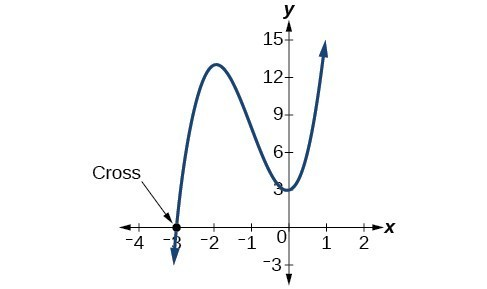Figure 2

Try It 4

Find the zeros of $f\left(x\right)=2{x}^{3}+5{x}^{2}-11x+4$.

Solution

Use the Linear Factorization Theorem to find polynomials with given zeros

A vital implication of the Fundamental Theorem of Algebra, as we stated above, is that a polynomial function of degree n will have n zeros in the set of complex numbers, if we allow for multiplicities. This means that we can factor the polynomial function into n factors. The Linear Factorization Theorem tells us that a polynomial function will have the same number of factors as its degree, and that each factor will be in the form (x – c), where c is a complex number.

Let f be a polynomial function with real coefficients, and suppose $a+bi\text{, }b\ne 0$, is a zero of $f\left(x\right)$. Then, by the Factor Theorem, $x-\left(a+bi\right)$ is a factor of $f\left(x\right)$. For f to have real coefficients, $x-\left(a-bi\right)$ must also be a factor of $f\left(x\right)$. This is true because any factor other than $x-\left(a-bi\right)$, when multiplied by $x-\left(a+bi\right)$, will leave imaginary components in the product. Only multiplication with conjugate pairs will eliminate the imaginary parts and result in real coefficients. In other words, if a polynomial function f with real coefficients has a complex zero $a+bi$, then the complex conjugate $a-bi$ must also be a zero of $f\left(x\right)$. This is called the Complex Conjugate Theorem.

A General Note: Complex Conjugate Theorem

According to the Linear Factorization Theorem, a polynomial function will have the same number of factors as its degree, and each factor will be in the form $\left(x-c\right)$, where c is a complex number.

If the polynomial function f has real coefficients and a complex zero in the form $a+bi$, then the complex conjugate of the zero, $a-bi$, is also a zero.

How To: Given the zeros of a polynomial function $f$ and a point $\left(c\text{, }f(c)\right)$ on the graph of $f$, use the Linear Factorization Theorem to find the polynomial function.

1. Use the zeros to construct the linear factors of the polynomial.
2. Multiply the linear factors to expand the polynomial.
3. Substitute $\left(c,f\left(c\right)\right)$ into the function to determine the leading coefficient.
4. Simplify.

Example 7: Using the Linear Factorization Theorem to Find a Polynomial with Given Zeros

Find a fourth degree polynomial with real coefficients that has zeros of –3, 2, i, such that $f\left(-2\right)=100$.

Solution

Because $x=i$ is a zero, by the Complex Conjugate Theorem $x=-i$ is also a zero. The polynomial must have factors of $\left(x+3\right),\left(x - 2\right),\left(x-i\right)$, and $\left(x+i\right)$. Since we are looking for a degree 4 polynomial, and now have four zeros, we have all four factors. Let’s begin by multiplying these factors.

$\begin{cases}f\left(x\right)=a\left(x+3\right)\left(x - 2\right)\left(x-i\right)\left(x+i\right)\\ f\left(x\right)=a\left({x}^{2}+x - 6\right)\left({x}^{2}+1\right)\\ f\left(x\right)=a\left({x}^{4}+{x}^{3}-5{x}^{2}+x - 6\right)\end{cases}$

We need to find a to ensure $f\left(-2\right)=100$. Substitute $x=-2$ and $f\left(2\right)=100$
into $f\left(x\right)$.

$\begin{cases}100=a\left({\left(-2\right)}^{4}+{\left(-2\right)}^{3}-5{\left(-2\right)}^{2}+\left(-2\right)-6\right)\hfill \\ 100=a\left(-20\right)\hfill \\ -5=a\hfill \end{cases}$

So the polynomial function is

$f\left(x\right)=-5\left({x}^{4}+{x}^{3}-5{x}^{2}+x - 6\right)$

or

$f\left(x\right)=-5{x}^{4}-5{x}^{3}+25{x}^{2}-5x+30$

Analysis of the Solution

We found that both i and –i were zeros, but only one of these zeros needed to be given. If i is a zero of a polynomial with real coefficients, then –i must also be a zero of the polynomial because –i is the complex conjugate of i.

Q & A

If 2 + 3i were given as a zero of a polynomial with real coefficients, would 2 – 3i also need to be a zero?

Yes. When any complex number with an imaginary component is given as a zero of a polynomial with real coefficients, the conjugate must also be a zero of the polynomial.

Try It 5

Find a third degree polynomial with real coefficients that has zeros of 5 and –2i such that $f\left(1\right)=10$.

Solution

Use Descartes’ Rule of Signs

There is a straightforward way to determine the possible numbers of positive and negative real zeros for any polynomial function. If the polynomial is written in descending order, Descartes’ Rule of Signs tells us of a relationship between the number of sign changes in $f\left(x\right)$ and the number of positive real zeros. For example, the polynomial function below has one sign change.

This tells us that the function must have 1 positive real zero.

There is a similar relationship between the number of sign changes in $f\left(-x\right)$ and the number of negative real zeros.

In this case, $f\left(\mathrm{-x}\right)$ has 3 sign changes. This tells us that $f\left(x\right)$ could have 3 or 1 negative real zeros.

A General Note: Descartes’ Rule of Signs

According to Descartes’ Rule of Signs, if we let $f\left(x\right)={a}_{n}{x}^{n}+{a}_{n - 1}{x}^{n - 1}+…+{a}_{1}x+{a}_{0}$ be a polynomial function with real coefficients:

• The number of positive real zeros is either equal to the number of sign changes of $f\left(x\right)$ or is less than the number of sign changes by an even integer.
• The number of negative real zeros is either equal to the number of sign changes of $f\left(-x\right)$ or is less than the number of sign changes by an even integer.

Example 7: Using Descartes’ Rule of Signs

Use Descartes’ Rule of Signs to determine the possible numbers of positive and negative real zeros for $f\left(x\right)=-{x}^{4}-3{x}^{3}+6{x}^{2}-4x - 12$.

Solution

Begin by determining the number of sign changes.

There are two sign changes, so there are either 2 or 0 positive real roots. Next, we examine $f\left(-x\right)$ to determine the number of negative real roots.

$\begin{cases}f\left(-x\right)=-{\left(-x\right)}^{4}-3{\left(-x\right)}^{3}+6{\left(-x\right)}^{2}-4\left(-x\right)-12\hfill \\ f\left(-x\right)=-{x}^{4}+3{x}^{3}+6{x}^{2}+4x - 12\hfill \end{cases}$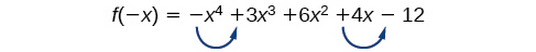Again, there are two sign changes, so there are either 2 or 0 negative real roots.

There are four possibilities, as we can see below.

Positive Real
Zeros
Negative Real
Zeros
Complex
Zeros
Total
Zeros
2 2 0 4
2 0 2 4
0 2 2 4
0 0 4 4

Analysis of the Solution

We can confirm the numbers of positive and negative real roots by examining a graph of the function. We can see from the graph in Figure 3 that the function has 0 positive real roots and 2 negative real roots.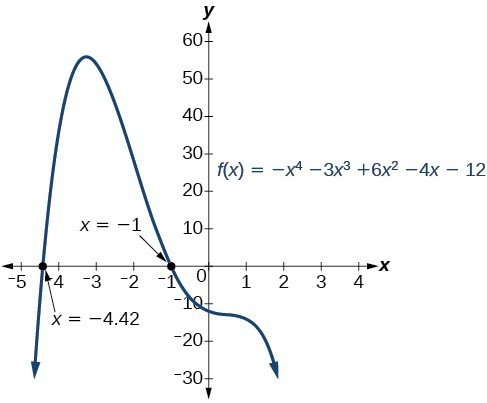Figure 3

Try It 6

Use Descartes’ Rule of Signs to determine the maximum possible numbers of positive and negative real zeros for $f\left(x\right)=2{x}^{4}-10{x}^{3}+11{x}^{2}-15x+12$. Use a graph to verify the numbers of positive and negative real zeros for the function.

Solution

Solve real-world applications of polynomial equations

We have now introduced a variety of tools for solving polynomial equations. Let’s use these tools to solve the bakery problem from the beginning of the section.

Example 8: Solving Polynomial Equations

A new bakery offers decorated sheet cakes for children’s birthday parties and other special occasions. The bakery wants the volume of a small cake to be 351 cubic inches. The cake is in the shape of a rectangular solid. They want the length of the cake to be four inches longer than the width of the cake and the height of the cake to be one-third of the width. What should the dimensions of the cake pan be?

Solution

Begin by writing an equation for the volume of the cake. The volume of a rectangular solid is given by $V=lwh$. We were given that the length must be four inches longer than the width, so we can express the length of the cake as $l=w+4$. We were given that the height of the cake is one-third of the width, so we can express the height of the cake as $h=\frac{1}{3}w$. Let’s write the volume of the cake in terms of width of the cake.

$\begin{cases}V=\left(w+4\right)\left(w\right)\left(\frac{1}{3}w\right)\\ V=\frac{1}{3}{w}^{3}+\frac{4}{3}{w}^{2}\end{cases}$

Substitute the given volume into this equation.

$\begin{cases}\text{ }351=\frac{1}{3}{w}^{3}+\frac{4}{3}{w}^{2}\hfill & \text{Substitute 351 for }V.\hfill \\ 1053={w}^{3}+4{w}^{2}\hfill & \text{Multiply both sides by 3}.\hfill \\ \text{ }0={w}^{3}+4{w}^{2}-1053 \hfill & \text{Subtract 1053 from both sides}.\hfill \end{cases}$

Descartes’ rule of signs tells us there is one positive solution. The Rational Zero Theorem tells us that the possible rational zeros are $\pm 3,\pm 9,\pm 13,\pm 27,\pm 39,\pm 81,\pm 117,\pm 351$, and $\pm 1053$. We can use synthetic division to test these possible zeros. Only positive numbers make sense as dimensions for a cake, so we need not test any negative values. Let’s begin by testing values that make the most sense as dimensions for a small sheet cake. Use synthetic division to check $x=1$.

Since 1 is not a solution, we will check $x=3$.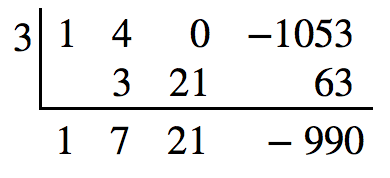Since 3 is not a solution either, we will test $x=9$.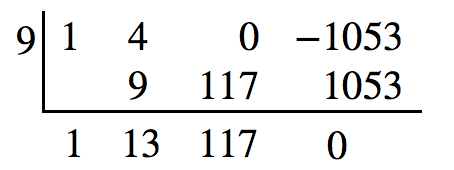Synthetic division gives a remainder of 0, so 9 is a solution to the equation. We can use the relationships between the width and the other dimensions to determine the length and height of the sheet cake pan.

$l=w+4=9+4=13\text{ and }h=\frac{1}{3}w=\frac{1}{3}\left(9\right)=3$

The sheet cake pan should have dimensions 13 inches by 9 inches by 3 inches.

Try It 7

A shipping container in the shape of a rectangular solid must have a volume of 84 cubic meters. The client tells the manufacturer that, because of the contents, the length of the container must be one meter longer than the width, and the height must be one meter greater than twice the width. What should the dimensions of the container be?

Solution

Key Concepts

• To find $f\left(k\right)$, determine the remainder of the polynomial $f\left(x\right)$ when it is divided by $x-k$.
• k is a zero of $f\left(x\right)$ if and only if $\left(x-k\right)$ is a factor of $f\left(x\right)$.
• Each rational zero of a polynomial function with integer coefficients will be equal to a factor of the constant term divided by a factor of the leading coefficient.
• When the leading coefficient is 1, the possible rational zeros are the factors of the constant term.
• Synthetic division can be used to find the zeros of a polynomial function.
• According to the Fundamental Theorem, every polynomial function has at least one complex zero.
• Every polynomial function with degree greater than 0 has at least one complex zero.
• Allowing for multiplicities, a polynomial function will have the same number of factors as its degree. Each factor will be in the form $\left(x-c\right)$, where c is a complex number.
• The number of positive real zeros of a polynomial function is either the number of sign changes of the function or less than the number of sign changes by an even integer.
• The number of negative real zeros of a polynomial function is either the number of sign changes of $f\left(-x\right)$ or less than the number of sign changes by an even integer.
• Polynomial equations model many real-world scenarios. Solving the equations is easiest done by synthetic division.

Glossary

Descartes’ Rule of Signs
a rule that determines the maximum possible numbers of positive and negative real zeros based on the number of sign changes of $f\left(x\right)$ and $f\left(-x\right)$
Factor Theorem
k is a zero of polynomial function $f\left(x\right)$ if and only if $\left(x-k\right)$ is a factor of $f\left(x\right)$
Fundamental Theorem of Algebra
a polynomial function with degree greater than 0 has at least one complex zero
Linear Factorization Theorem
allowing for multiplicities, a polynomial function will have the same number of factors as its degree, and each factor will be in the form $\left(x-c\right)$, where c is a complex number
Rational Zero Theorem
the possible rational zeros of a polynomial function have the form $\frac{p}{q}$ where p is a factor of the constant term and q is a factor of the leading coefficient.
Remainder Theorem
if a polynomial $f\left(x\right)$ is divided by $x-k$, then the remainder is equal to the value $f\left(k\right)$

Section Exercises

1. Describe a use for the Remainder Theorem.

2. Explain why the Rational Zero Theorem does not guarantee finding zeros of a polynomial function.

3. What is the difference between rational and real zeros?

4. If Descartes’ Rule of Signs reveals a no change of signs or one sign of changes, what specific conclusion can be drawn?

5. If synthetic division reveals a zero, why should we try that value again as a possible solution?

For the following exercises, use the Remainder Theorem to find the remainder.

6. $\left({x}^{4}-9{x}^{2}+14\right)\div \left(x - 2\right)$

7. $\left(3{x}^{3}-2{x}^{2}+x - 4\right)\div \left(x+3\right)$

8. $\left({x}^{4}+5{x}^{3}-4x - 17\right)\div \left(x+1\right)$

9. $\left(-3{x}^{2}+6x+24\right)\div \left(x - 4\right)$

10. $\left(5{x}^{5}-4{x}^{4}+3{x}^{3}-2{x}^{2}+x - 1\right)\div \left(x+6\right)$

11. $\left({x}^{4}-1\right)\div \left(x - 4\right)$

12. $\left(3{x}^{3}+4{x}^{2}-8x+2\right)\div \left(x - 3\right)$

13. $\left(4{x}^{3}+5{x}^{2}-2x+7\right)\div \left(x+2\right)$

For the following exercises, use the Factor Theorem to find all real zeros for the given polynomial function and one factor.

14. $f\left(x\right)=2{x}^{3}-9{x}^{2}+13x - 6;\text{ }x - 1$

15. $f\left(x\right)=2{x}^{3}+{x}^{2}-5x+2;\text{ }x+2$

16. $f\left(x\right)=3{x}^{3}+{x}^{2}-20x+12;\text{ }x+3$

17. $f\left(x\right)=2{x}^{3}+3{x}^{2}+x+6;x+2$

18. $f\left(x\right)=-5{x}^{3}+16{x}^{2}-9;x - 3$

19. ${x}^{3}+3{x}^{2}+4x+12;x+3$

20. $4{x}^{3}-7x+3;x - 1$

21. $2{x}^{3}+5{x}^{2}-12x - 30,\text{ }2x+5$

For the following exercises, use the Rational Zero Theorem to find all real zeros.

22. ${x}^{3}-3{x}^{2}-10x+24=0$

23. $2{x}^{3}+7{x}^{2}-10x - 24=0$

24. ${x}^{3}+2{x}^{2}-9x - 18=0$

25. ${x}^{3}+5{x}^{2}-16x - 80=0$

26. ${x}^{3}-3{x}^{2}-25x+75=0$

27. $2{x}^{3}-3{x}^{2}-32x - 15=0$

28. $2{x}^{3}+{x}^{2}-7x - 6=0$

29. $2{x}^{3}-3{x}^{2}-x+1=0$

30. $3{x}^{3}-{x}^{2}-11x - 6=0$

31. $2{x}^{3}-5{x}^{2}+9x - 9=0$

32. $2{x}^{3}-3{x}^{2}+4x+3=0$

33. ${x}^{4}-2{x}^{3}-7{x}^{2}+8x+12=0$

34. ${x}^{4}+2{x}^{3}-9{x}^{2}-2x+8=0$

35. $4{x}^{4}+4{x}^{3}-25{x}^{2}-x+6=0$

36. $2{x}^{4}-3{x}^{3}-15{x}^{2}+32x - 12=0$

37. ${x}^{4}+2{x}^{3}-4{x}^{2}-10x - 5=0$

38. $4{x}^{3}-3x+1=0$

39. $8{x}^{{}^{4}}+26{x}^{3}+39{x}^{2}+26x+6$

For the following exercises, find all complex solutions (real and non-real).

40. ${x}^{3}+{x}^{2}+x+1=0$

41. ${x}^{3}-8{x}^{2}+25x - 26=0$

42. ${x}^{3}+13{x}^{2}+57x+85=0$

43. $3{x}^{3}-4{x}^{2}+11x+10=0$

44. ${x}^{4}+2{x}^{3}+22{x}^{2}+50x - 75=0$

45. $2{x}^{3}-3{x}^{2}+32x+17=0$

For the following exercises, use Descartes’ Rule to determine the possible number of positive and negative solutions. Confirm with the given graph.

46. $f\left(x\right)={x}^{3}-1$

47. $f\left(x\right)={x}^{4}-{x}^{2}-1$

48. $f\left(x\right)={x}^{3}-2{x}^{2}-5x+6$

49. $f\left(x\right)={x}^{3}-2{x}^{2}+x - 1$

50. $f\left(x\right)={x}^{4}+2{x}^{3}-12{x}^{2}+14x - 5$

51. $f\left(x\right)=2{x}^{3}+37{x}^{2}+200x+300$

52. $f\left(x\right)={x}^{3}-2{x}^{2}-16x+32$

53. $f\left(x\right)=2{x}^{4}-5{x}^{3}-5{x}^{2}+5x+3$

54. $f\left(x\right)=2{x}^{4}-5{x}^{3}-14{x}^{2}+20x+8$

55. $f\left(x\right)=10{x}^{4}-21{x}^{2}+11$

For the following exercises, list all possible rational zeros for the functions.

56. $f\left(x\right)={x}^{4}+3{x}^{3}-4x+4$

57. $f\left(x\right)=2{x}^{{}^{3}}+3{x}^{2}-8x+5$

58. $f\left(x\right)=3{x}^{{}^{3}}+5{x}^{2}-5x+4$

59. $f\left(x\right)=6{x}^{4}-10{x}^{2}+13x+1$

60. $f\left(x\right)=4{x}^{5}-10{x}^{4}+8{x}^{3}+{x}^{2}-8$

For the following exercises, use your calculator to graph the polynomial function. Based on the graph, find the rational zeros. All real solutions are rational.

61. $f\left(x\right)=6{x}^{3}-7{x}^{2}+1$

62. $f\left(x\right)=4{x}^{3}-4{x}^{2}-13x - 5$

63. $f\left(x\right)=8{x}^{3}-6{x}^{2}-23x+6$

64. $f\left(x\right)=12{x}^{4}+55{x}^{3}+12{x}^{2}-117x+54$

65. $f\left(x\right)=16{x}^{4}-24{x}^{3}+{x}^{2}-15x+25$

For the following exercises, construct a polynomial function of least degree possible using the given information.

66. Real roots: –1, 1, 3 and $\left(2,f\left(2\right)\right)=\left(2,4\right)$

67. Real roots: –1 (with multiplicity 2 and 1) and $\left(2,f\left(2\right)\right)=\left(2,4\right)$

68. Real roots: –2, $\frac{1}{2}$ (with multiplicity 2) and $\left(-3,f\left(-3\right)\right)=\left(-3,5\right)$

69. Real roots: $-\frac{1}{2}$, 0, $\frac{1}{2}$ and $\left(-2,f\left(-2\right)\right)=\left(-2,6\right)$

70. Real roots: –4, –1, 1, 4 and $\left(-2,f\left(-2\right)\right)=\left(-2,10\right)$

For the following exercises, find the dimensions of the box described.

71. The length is twice as long as the width. The height is 2 inches greater than the width. The volume is 192 cubic inches.

72. The length, width, and height are consecutive whole numbers. The volume is 120 cubic inches.

73. The length is one inch more than the width, which is one inch more than the height. The volume is 86.625 cubic inches.

74. The length is three times the height and the height is one inch less than the width. The volume is 108 cubic inches.

75. The length is 3 inches more than the width. The width is 2 inches more than the height. The volume is 120 cubic inches.

For the following exercises, find the dimensions of the right circular cylinder described.

76. The radius is 3 inches more than the height. The volume is $16\pi$ cubic meters.

77. The height is one less than one half the radius. The volume is $72\pi$ cubic meters.

78. The radius and height differ by one meter. The radius is larger and the volume is $48\pi$ cubic meters.

79. The radius and height differ by two meters. The height is greater and the volume is $28.125\pi$
cubic meters.

80. The radius is $\frac{1}{3}$ meter greater than the height. The volume is $\frac{98}{9}\pi$
cubic meters.